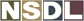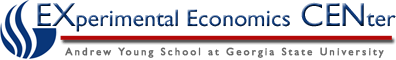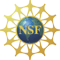Students / Subjects# Calculating GDP

To calculate GDP for a number of different goods national income accounting uses market prices.  For instance, if the economy were to produce eight onions and four pears and onions were sold for \$1.00 each and pears were sold for \$1.50 each, GDP would equal the total of the quantity of onions times its price and the quantity of pears times its price.  This would look like:

GDP = (Price of Onions x Quantity of Onions) + (Price of Pears x Quantity of Pears)

GDP = (\$1.00 x 8) + (\$1.50 x 4) = \$14.00

There are two principal ways of calculating GDP:

Expenditures Approach: GDP = C + I + G + (X-M)

and

Income Approach: NI = W + R + i + PR

The first focuses on total expenditures on goods and services produced in the period, while the second concentrates on the payments to the factors of production involved in those production activities within the period. In principle, the two approaches should result in the same GDP value since expenditure by one party is always an income for some other party. However, some discrepancies arise due to measurement errors.

### The two ways of calculating GDP: